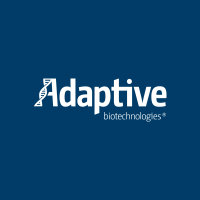11.52 USD -0.33 USD ( -2.78% )-

-

## FAQ

### What is Discount Rate?

Discount rate reflects the opportunity cost of investment (i.e. the return that could be earned on investment with similar risk).

### Cost of Equity Calculation

Cost of Equity
-
Rf
-
Beta
-
ERP
-

The Cost of Equity for Adaptive Biotechnologies Corp (NASDAQ:ADPT) calculated via CAPM (Capital Asset Pricing Model) is -.

WACC
-

Cost of Equity
-
Equity Weight
-

Cost of Debt
-
Debt Weight
-